# A mass weighing 4 kg is hooked to the end of a spring.

A mass weighing 4 kg is hooked to the end of a spring. The spring constant is 16 kg/meter.

Find the equation of motion and period of simple harmonic motion.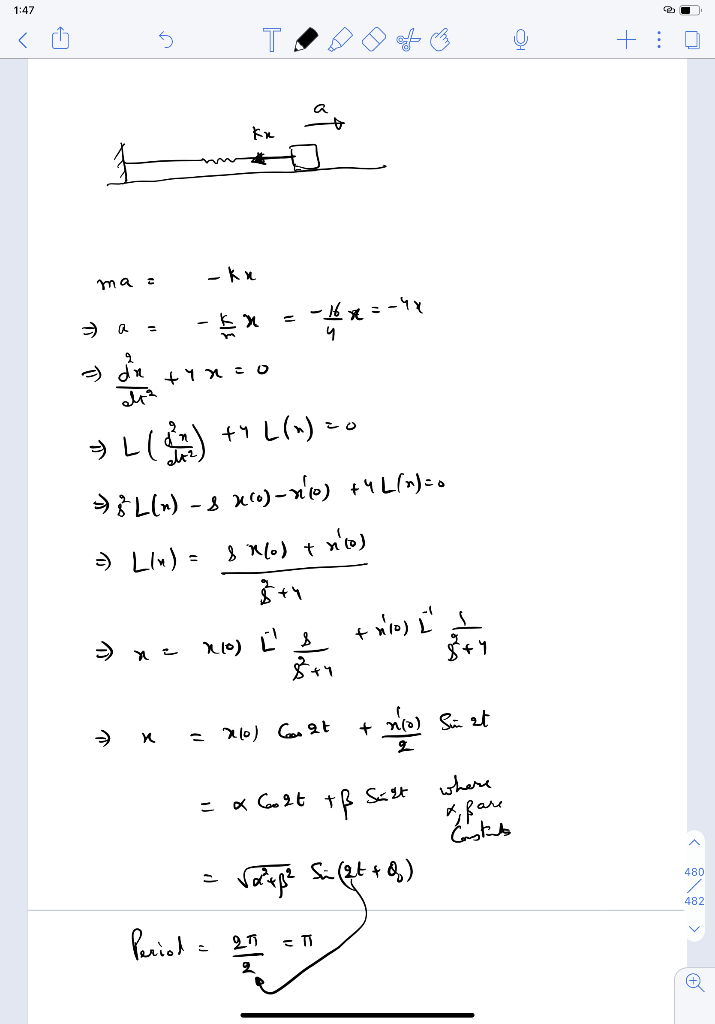#### Earn Coin

Coins can be redeemed for fabulous gifts.

Similar Homework Help Questions
• ### A horizontal mass-spring system consists of a 2 kg mass moving on a frictionless surface attached...

A horizontal mass-spring system consists of a 2 kg mass moving on a frictionless surface attached to a spring. The other end of the spring is attached to a wall. The mass is pulled and released. The resultant simple harmonic motion has a period of 5 s and it is observed that the maximum velocity of the mass is 0.3 m/s. a) Calculate the spring constant of the spring. (b) Calculate the amplitude of the motion. Sometime later, when the...

• ### Problems 1 and 2 A mass weighing 3/4 slug, attached to the end of a spring...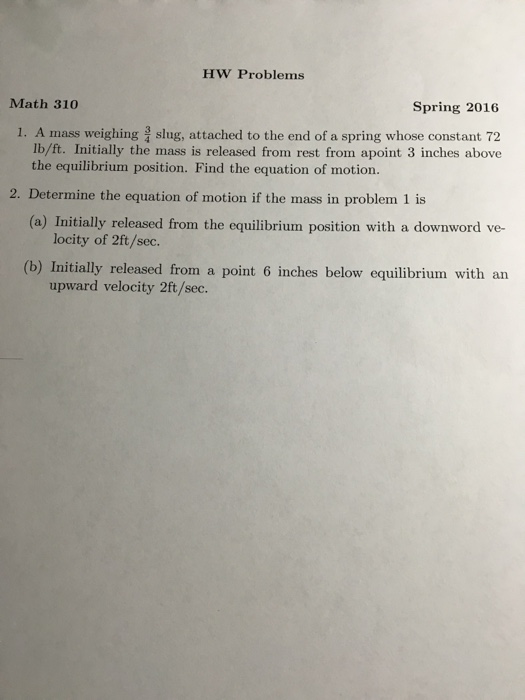Problems 1 and 2 A mass weighing 3/4 slug, attached to the end of a spring whose constant 72 lb/ft. Initially the mass is released from rest from a point 3 inches above the equilibrium position. Find the equation of motion. Determine the equation of motion if the mass in problem 1 is Initially released from the equilibrium position with a downword velocity of 2ft/sec. Initially released from a point 6 inches below equilibrium with an upward velocity 2ft/sec.

• ### 4. (15 pts) A mass weighing 8 pounds, attached to the end of a spring, stretches...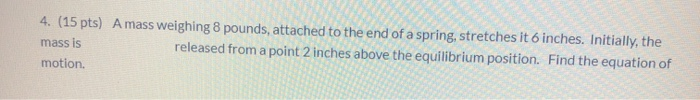4. (15 pts) A mass weighing 8 pounds, attached to the end of a spring, stretches it 6 inches. Initially, the mass is released from a point 2 inches above the equilibrium position. Find the equation of motion.

• ### A 0.50 kg mass oscillates in simple harmonic motion on a spring with a spring constant...

A 0.50 kg mass oscillates in simple harmonic motion on a spring with a spring constant of 210 N/m . Part A What is the period of the oscillation? Part B What is the frequency of the oscillation?

• ### A 0.8 kg mass attached to a vertical spring undergoes simple harmonic motion with a frequency...

A 0.8 kg mass attached to a vertical spring undergoes simple harmonic motion with a frequency of 0.5 Hz. a) What is the period of the motion and the spring constant? b) If the amplitude of oscillation is 10 cm and the mass starts at its lowest point at time zero, write the equation describing the displacement of the mass as a function of time and find the position of the mass at times 1, 2, 1.5 s, and 1.25...

• ### 10. A 1.5 kg mass is attached to the end set into simple harmonic motion wit...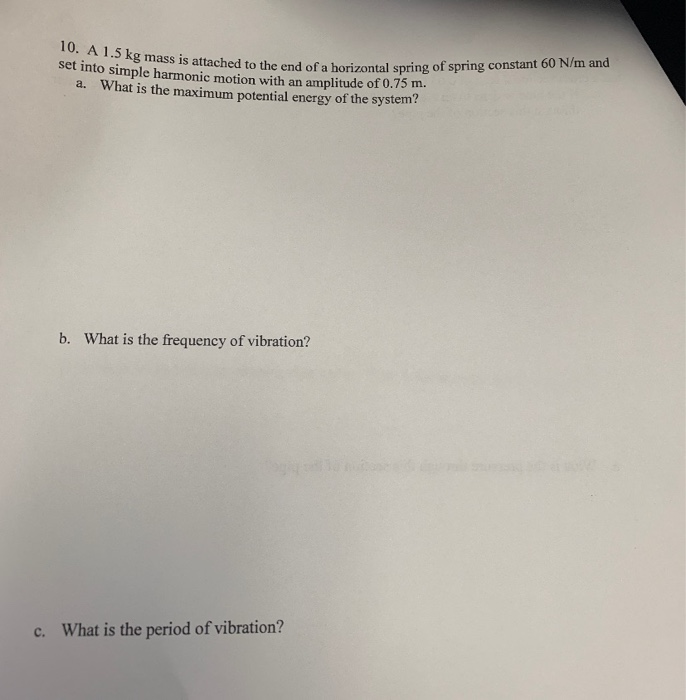10. A 1.5 kg mass is attached to the end set into simple harmonic motion wit ned to the end of a horizontal spring of spring constant 60 N/m and a. What is the maximum potential energy of the system? b. What is the frequency of vibration? c. What is the period of vibration?

• ### (a) Obtain the equation of motion for a 2.75-g mass, at the end of a perfectly...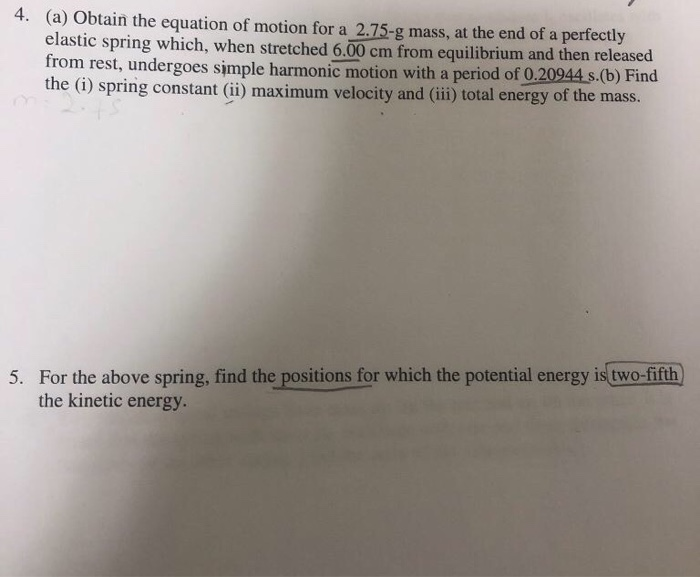(a) Obtain the equation of motion for a 2.75-g mass, at the end of a perfectly elastic spring which, when stretched 6.00 cm from equilibrium and then released from rest, undergoes simple harmonic motion with a period of 0.20944 s.(b) Find the (i) spring constant (ii) maximum velocity and (iii) total energy of the mass. 4. For the above spring, find the positions for which the potential energy is two-fifth the kinetic energy. 5.

• ### Part A: 10 points each (Questions 1-4 1. A block mass of 3 kg attached with a spring kg attached with a spring of s...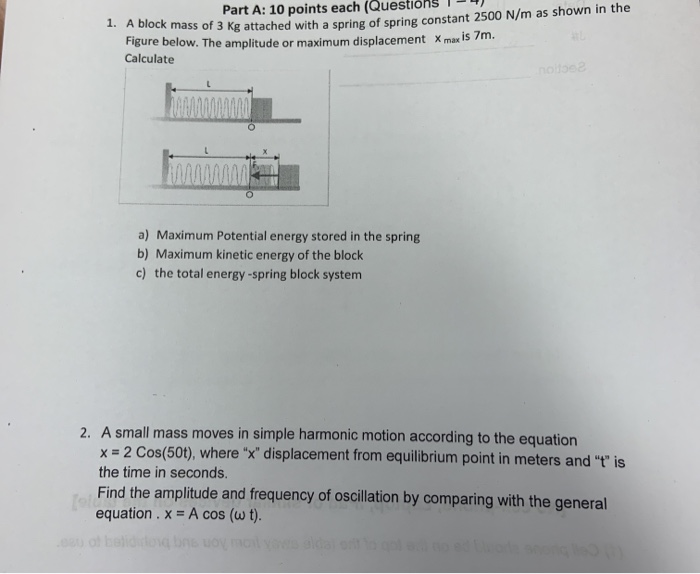Part A: 10 points each (Questions 1-4 1. A block mass of 3 kg attached with a spring kg attached with a spring of spring constant 2500 N/m as shown in the Figure below. The amplitude or maximum displacement X max is 7m. Calculate O a) Maximum Potential energy stored in the spring b) Maximum kinetic energy of the block c) the total energy-spring block system 2. A small mass moves in simple harmonic motion according to the equation x...

• ### A mass weighing 12 pounds stretches a spring 2 feet. The mass is initially released from...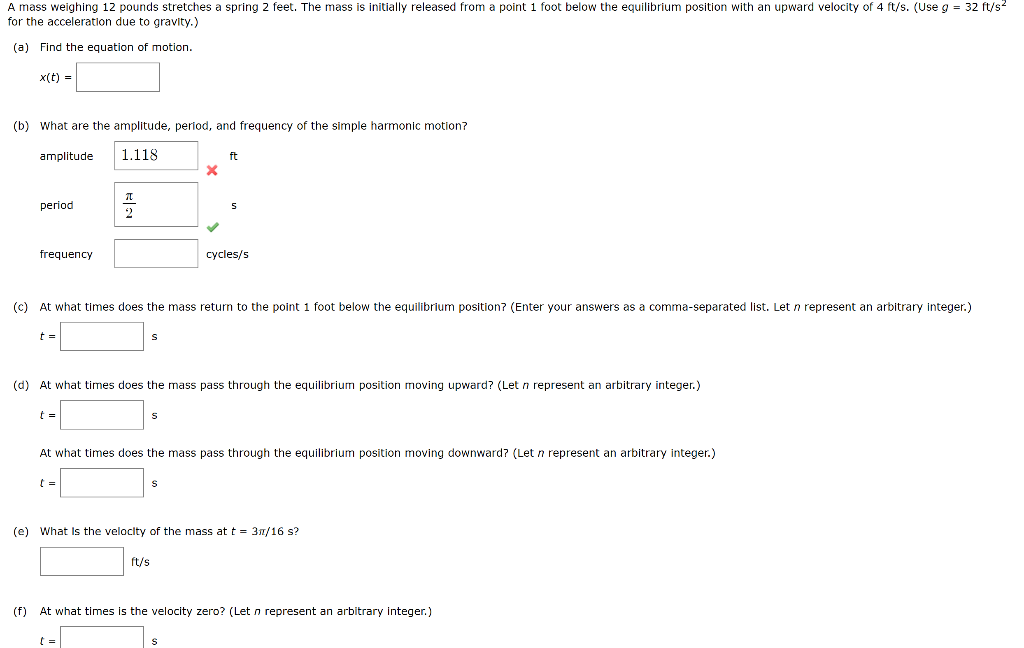A mass weighing 12 pounds stretches a spring 2 feet. The mass is initially released from a point 1 foot below the equilibrium position with an upward velocity of 4 ft/s. (Use g 32 ft/s for the acceleration due to gravity.) (a) Find the equation of motion x(t) (b) what are the amplitude, period, and frequency of the simple harmonic motion? amplitude1.118 ft period frequency cycles/s (c) At what times does the mass return to the point 1 foot below...

• ### 2. A block of unknown mass is attached to a spring with a spring constant of...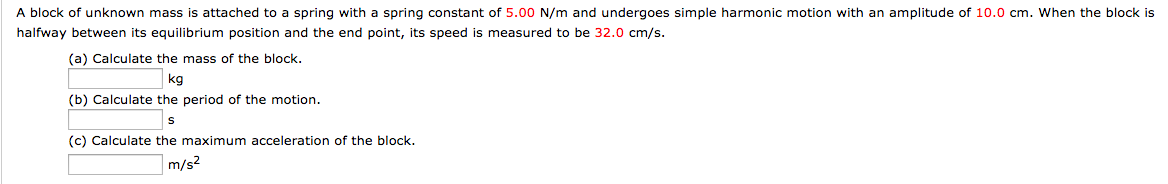2. A block of unknown mass is attached to a spring with a spring constant of 5.00 N/m and undergoes simple harmonic motion with an amplitude of 10.0 cm. When the block is halfway between its equilibrium position and the end point, its speed is measured to be 32.0 cm/s (a) Calculate the mass of the block (b) Calculate the period of the motion (c) Calculate the maximum acceleration of the block. kg m/s